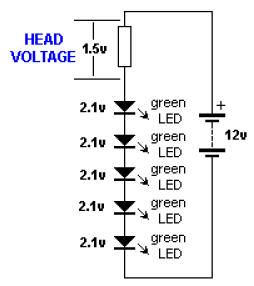Electronic

Now we turn our attention to the resistor.

As the supply-voltage increases, the voltage across the LED will be constant at 1.7v (for a red LED) and the excess voltage will be dropped across the resistor.

The supply can be any voltage from 2v to 12v or more. In this case, the resistor will drop 0.3v to 10.3v.

And the resistor is called the CURRENT-LIMIT resistor.

The following diagram shows HEAD VOLTAGE:The voltage dropped across this resistor, combined with the current, constitutes wasted energy and should be kept to a minimum, but a small HEAD VOLTAGE is not advisable (such as 0.5v).

The head voltage should be a minimum of 1.5v - and this only applies if the supply is fixed.

The head voltage depends on the supply voltage.

If the supply is fixed and guaranteed not to increase or fall, the head voltage can be small (1.5v minimum).

But most supplies are derived from batteries and the voltage will drop as the cells are used.

Here is an example of a problem:

Supply voltage:

12v 7 red LEDs in series = 11.9v

Dropper resistor = 0.1v As soon as the supply drops to 11.8v, no LEDs will be illuminated.

(Sometimes the LEDs will illuminate because some LEDs will have a characteristic voltage that is slightly less than 1.7v and some will illuminate when the voltage is lower than 1.6v - but the brightness will reduce considerably.)

Example 2:

Supply voltage 12v 5 green LEDs in series @ 2.1v = 10.5v

Dropper resistor = 1.5v

The battery voltage can drop to 10.5v But let's look at the situation more closely.

Suppose the current @ 12v = 25mA.

As the voltage drops, the current will drop. At 11.5v, the current will be 17mA At 11v, the current will be 9mA At 10.5v, the current will be zero You can see the workable supply drop is only about 1v.

Many batteries drop 1v and still have over 80% of their energy remaining.

That's why you need to design your circuit to have a large HEAD VOLTAGE.

A large Head Voltage is also needed when a plug-pack (wall wart) is used.

These devices consist of a transformer, set of diodes and an electrolytic.

The voltage marked on the unit is the voltage it will deliver when fully loaded.

It may be 200mA, 300mA or 500mA.

When this current is delivered, the voltage will be 9v or 12v.

But if the current is less than the rated current, the output voltage will be higher.

It may be 1v, 2v or even 5v higher.

This is one of the characteristics of a cheap transformer.

A cheap transformer has very poor regulation, so to deliver 12v @ 500mA, the transformer produces a higher voltage on no-load and the voltage drops as the current increases.

You need to allow for this extra voltage when using a plug-pack so the LEDs do not take more than 20mA to 25mA.

Roger Mew contacted me asking for some suitable resistances for the HEAD VOLTAGE resistor.

Here is a list:

For 25mA current:

Use 56R for 1.5v drop.

Use 82R for 2v drop

Use 120R for 3v drop

Use 150R for 4v drop

Use 180R for about 5v drop

Recherche personnalisée